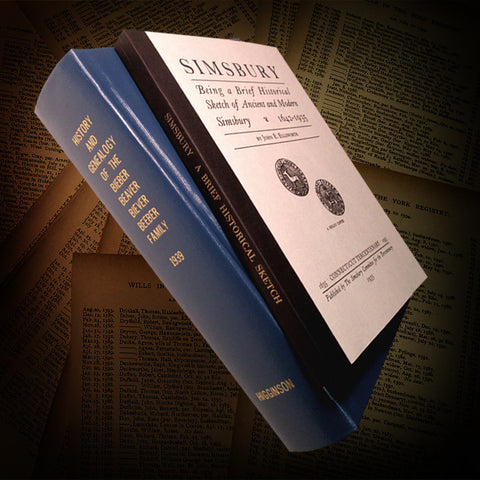## CHEMICAL REACTION EQUILIBRIUM THEORY - 3rd Edition (Hardcover)

• 11000

By Barry D. Josephs, 248 pages, (Hardcover Only)

Copyright 2015.  Published by Higginson Book Co. LLC.

Description:
Pathways to a better understanding of reaction equilibrium. Derivation procedures employing Gibbs energy and Helmholtz energy functions are applied to ideal and non-ideal mixtures to determine reaction equilibrium for gaseous and liquid phase systems. Derivations show rate of change of Gibbs energy as the reaction proceeds to equilibrium. Derivations calculate the equilibrium constant to determine composition at the point of equilibrium. Underlying thermodynamic principles utilizing the first, second, and third laws of thermodynamics are discussed. The effects of elevated pressure and temperature derived. Equilibrium for simultaneous reactions determined. Equation derived for maximum non-mechanical work possible as the reaction proceeds towards equilibrium. Derivation of the Nernst equation for determination of cell voltage presented. Illustrative examples, graphical presentations, References and detailed Appendix included.

We Also Recommend